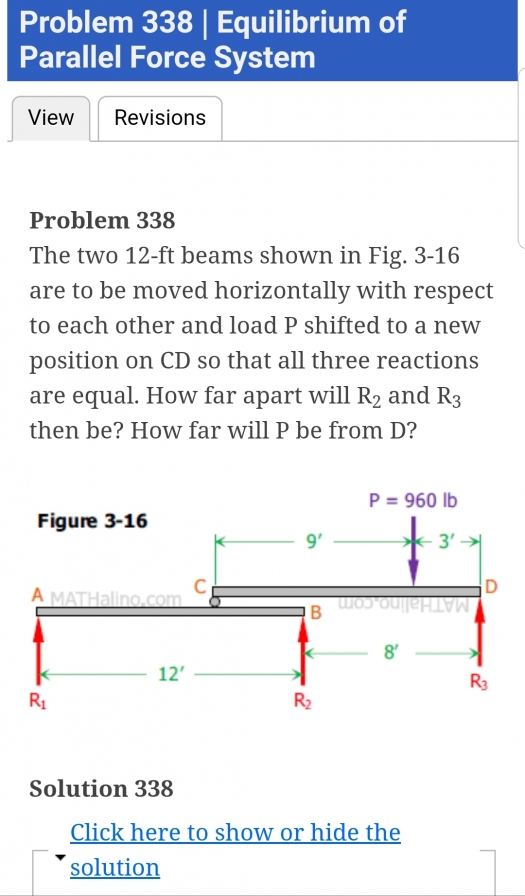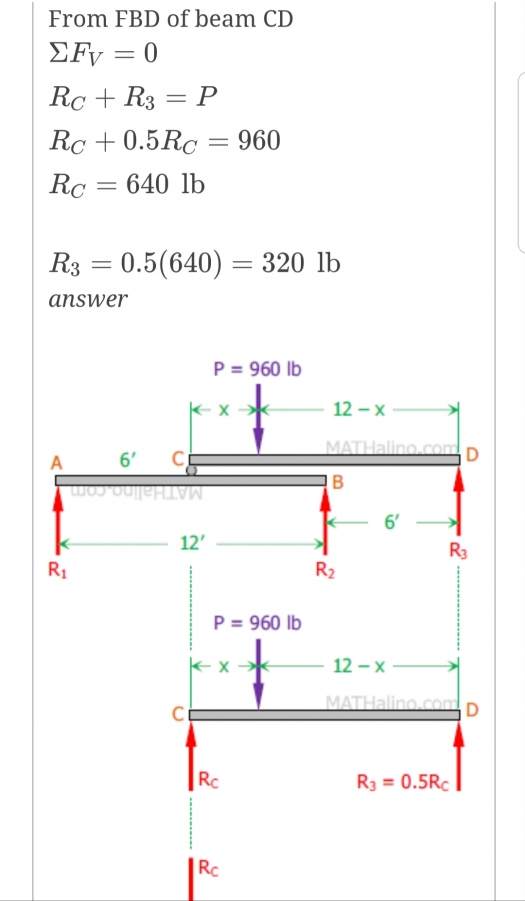# Statics

Good day sir. I just want to ask about Problem 338. Why is R3 equal to half of Rc?Tags:### Because R1 = R2 = R3. For R1

Because R1 = R2 = R3. For R1 and R2 to be equal, it should be half the RC.

• Mathematics inside the configured delimiters is rendered by MathJax. The default math delimiters are $$...$$ and $...$ for displayed mathematics, and $...$ and $...$ for in-line mathematics.